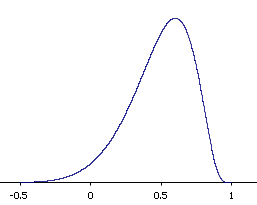# 9.7: Sampling Distribution of Pearson's r

•• David Lane
• Rice University
$$\newcommand{\vecs}{\overset { \rightharpoonup} {\mathbf{#1}} }$$ $$\newcommand{\vecd}{\overset{-\!-\!\rightharpoonup}{\vphantom{a}\smash {#1}}}$$$$\newcommand{\id}{\mathrm{id}}$$ $$\newcommand{\Span}{\mathrm{span}}$$ $$\newcommand{\kernel}{\mathrm{null}\,}$$ $$\newcommand{\range}{\mathrm{range}\,}$$ $$\newcommand{\RealPart}{\mathrm{Re}}$$ $$\newcommand{\ImaginaryPart}{\mathrm{Im}}$$ $$\newcommand{\Argument}{\mathrm{Arg}}$$ $$\newcommand{\norm}{\| #1 \|}$$ $$\newcommand{\inner}{\langle #1, #2 \rangle}$$ $$\newcommand{\Span}{\mathrm{span}}$$ $$\newcommand{\id}{\mathrm{id}}$$ $$\newcommand{\Span}{\mathrm{span}}$$ $$\newcommand{\kernel}{\mathrm{null}\,}$$ $$\newcommand{\range}{\mathrm{range}\,}$$ $$\newcommand{\RealPart}{\mathrm{Re}}$$ $$\newcommand{\ImaginaryPart}{\mathrm{Im}}$$ $$\newcommand{\Argument}{\mathrm{Arg}}$$ $$\newcommand{\norm}{\| #1 \|}$$ $$\newcommand{\inner}{\langle #1, #2 \rangle}$$ $$\newcommand{\Span}{\mathrm{span}}$$

Learning Objectives

• Transform $$r$$ to $$z'$$
• Calculate the probability of obtaining an $$r$$ above a specified value

Assume that the correlation between quantitative and verbal SAT scores in a given population is $$0.60$$. In other words, $$\rho =0.60$$. If $$12$$ students were sampled randomly, the sample correlation, $$r$$, would not be exactly equal to $$0.60$$. Naturally different samples of $$12$$ students would yield different values of $$r$$. The distribution of values of $$r$$ after repeated samples of $$12$$ students is the sampling distribution of $$r$$.

The shape of the sampling distribution of $$r$$ for the above example is shown in Figure $$\PageIndex{1}$$. You can see that the sampling distribution is not symmetric: it is negatively skewed. The reason for the skew is that $$r$$ cannot take on values greater than $$1.0$$ and therefore the distribution cannot extend as far in the positive direction as it can in the negative direction. The greater the value of $$\rho$$, the more pronounced the skew.Figure $$\PageIndex{1}$$: The sampling distribution of $$r$$ for $$N = 12$$ and $$\rho =0.60$$

Figure $$\PageIndex{2}$$ shows the sampling distribution for $$\rho =0.90$$. This distribution has a very short positive tail and a long negative tail.Figure $$\PageIndex{1}$$: The sampling distribution of $$r$$ for $$N = 12$$ and $$\rho =0.90$$

Referring back to the SAT example, suppose you wanted to know the probability that in a sample of $$12$$ students, the sample value of $$r$$ would be $$0.75$$ or higher. You might think that all you would need to know to compute this probability is the mean and standard error of the sampling distribution of $$r$$. However, since the sampling distribution is not normal, you would still not be able to solve the problem. Fortunately, the statistician Fisher developed a way to transform $$r$$ to a variable that is normally distributed with a known standard error. The variable is called $$z'$$ and the formula for the transformation is given below.

$z' = 0.5\: \ln \frac{1+r}{1-r}$

The details of the formula are not important here since normally you will use either a table, Pearson r to Fisher z' or calculator to do the transformation. What is important is that $$z'$$ is normally distributed and has a standard error of

$\frac{1}{\sqrt{N-3}}$

where $$N$$ is the number of pairs of scores.

Example $$\PageIndex{1}$$

Let's return to the question of determining the probability of getting a sample correlation of $$0.75$$ or above in a sample of $$12$$ from a population with a correlation of $$0.60$$.

Solution

The first step is to convert both $$0.60$$ and $$0.75$$ to their $$z'$$ values, which are $$0.693$$ and $$0.973$$, respectively.

The standard error of $$z'$$ for $$N = 12$$ is $$0.333$$.

Therefore the question is reduced to the following: given a normal distribution with a mean of $$0.693$$ and a standard deviation of $$0.333$$, what is the probability of obtaining a value of $$0.973$$ or higher?

The answer can be found directly from the applet "Calculate Area for a given X" to be $$0.20$$.

Alternatively, you could use the formula:

$z = \frac{X - \mu }{\sigma } = \frac{0.973 - 0.693}{0.333} = 0.841$

and use a table to find that the area above $$0.841$$ is $$0.20$$.

This page titled 9.7: Sampling Distribution of Pearson's r is shared under a Public Domain license and was authored, remixed, and/or curated by David Lane via source content that was edited to the style and standards of the LibreTexts platform; a detailed edit history is available upon request.